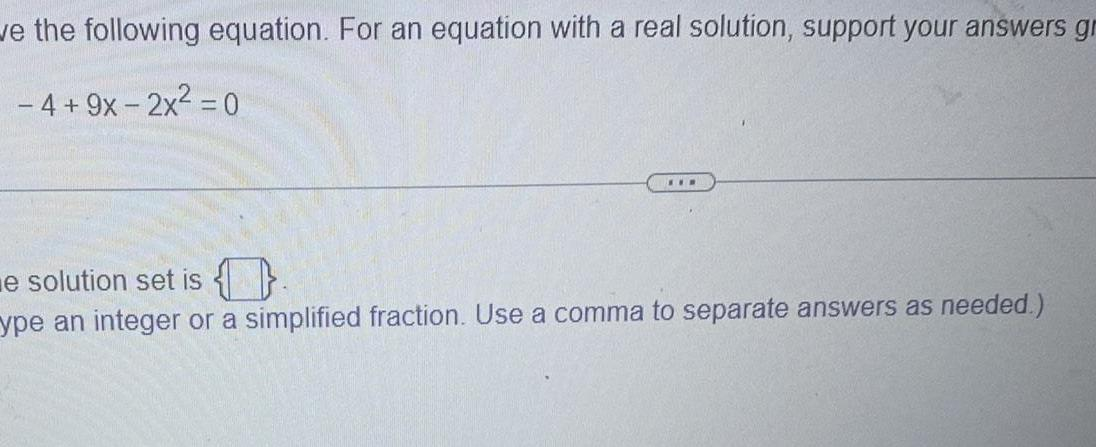Question:

# ve the following equation For an equation with a real

Last updated: 9/19/2023ve the following equation For an equation with a real solution support your answers gr 4 9x 2x 0 EER e solution set is ype an integer or a simplified fraction Use a comma to separate answers as needed